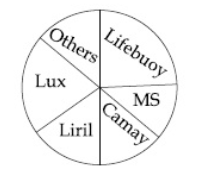Question 10

# The total volume of soaps sold is 680 lakhs units. The volume of Lux sold is 136 lakh units. If the percentage of Lifebuoy sold is 30%, Camay 10%, Liril 15% and Mysore Sandal 10%, answer the following questions.What is the market share of others in percentage points?

Solution

Given, Total volume of soaps sold = 680 lakh units.

Volume of Lux sold = 136 lakh units.

$$\Rightarrow$$ Percentage of Lux sold = $$\frac{136}{680}\times 100$$ = 20%

Also given,

percentage of Lifebuoy sold is 30%,

percentage of Camay 10%,

percentage of Liril 15% and

percentage of Mysore Sandal 10%

From the pie chart

The percentage of Lux + Lifeboy + Camay + Liril + Mysore Sandal + Others  sold = 100%

$$\Rightarrow$$ The market share of others = 100 - 20 - 30 - 10 - 15 - 10 = 15%